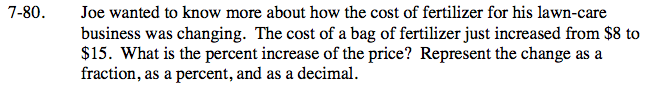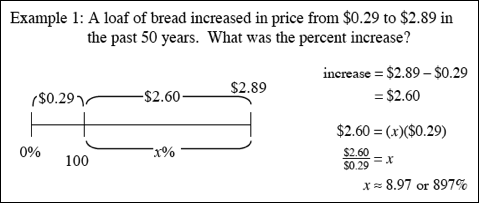Home > CC2MN > Chapter cc27 > Lesson cc27.1.7 > Problem7-80

7-80.

Joe wanted to know more about how the cost of fertilizer for his lawn-care business was changing. The cost of a bag of fertilizer just increased from $8 to$15. What is the percent increase of the price? Represent the change as a fraction, as a percent, and as a decimal. Homework Help ✎Refer to the example at right from the Math Notes box in this lesson for help with percent increases.

Increase = $7$7 = (x)(\$8)

$\frac{7}{8}=0.875, \;87.5\%$